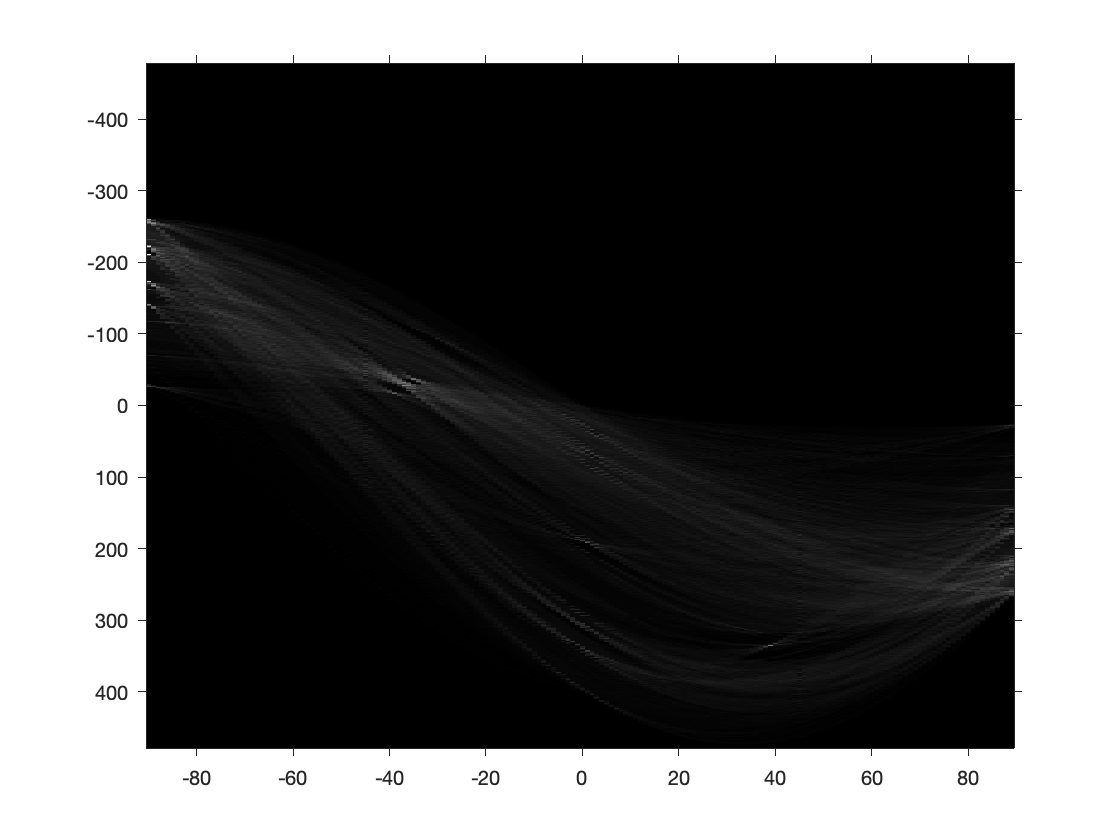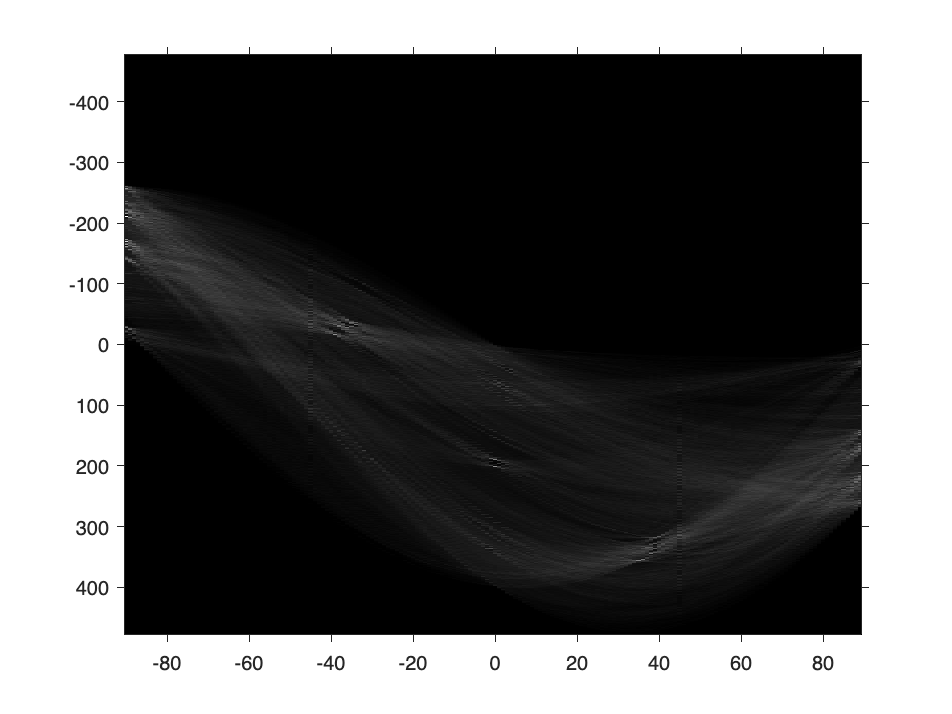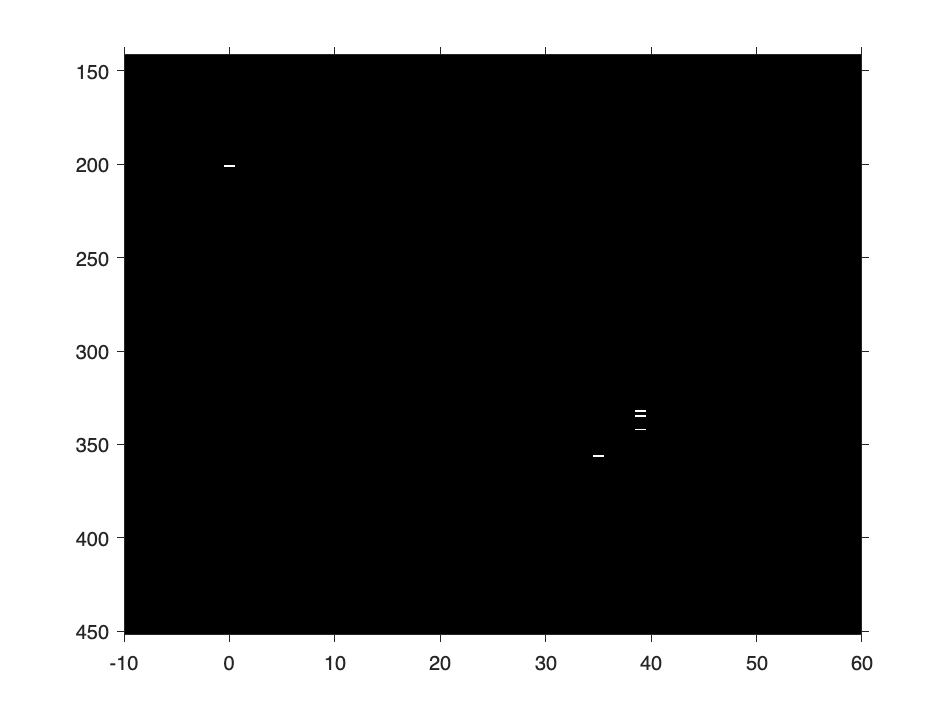# Find all the highest pixel values using ismember

Today's post shows how to use ismember to conveniently locate all of the highest pixel values in an image. For example, in the following Hough transform image, what are the 20 highest values, and where are all the pixels that have those values?I got the idea from some example code written by Image Processing Toolbox writer Megan Mancuso. Megan says she got the idea to use ismember this way from Ahmed's answer on MATLAB Answers.
Here's where the Hough transform image above comes from.
imshow(A)bw = edge(rgb2gray(A),"canny");
imshow(bw)[H,T,R] = hough(bw);
imshow(H,[],XData=T,YData=R)
daspect auto
axis onNow let's sort to figure out the highest 20 values of H.
sorted_H_values = sort(H(:),"descend");
highest_H_values = sorted_H_values(1:20)
highest_H_values = 20×1
293 250 209 205 182 177 173 172 171 168
Finally, I'll use ismember to compute a binary image showing all the elements of H that have one of those 20 highest values. (And I'll use xlim and ylim to zoom in so that we can see a few of those elements clearly.)